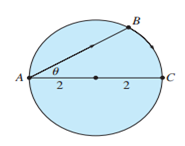Chapter 3.7, Problem 50E

Chapter
Section
Textbook Problem

# A woman at a point A on the shore of a circular lake with radius 2 mi wants to arrive at the point C diametrically opposite A on the other side of the lake in the shortest possible time (see the figure). She can walk at the rate of 4 mi/h and row a boat at 2 mi/h. How should she proceed?To determine

To find:

How should a woman proceed?

Explanation

1) Concept:

A critical number of a function f   is a number c in the domain of f  Such that either  f'c=0 or f'c does not exist.

Cosine rule:

For a triangle with angles A, B and C with corresponding opposite sides a, b and c, the cosine rule can be written as a2=b2+c2-2bc·cosA

2) Given:

A woman can walk at the rate of 4 mi/h and 2 mi/h.

3) Calculation:

By joining center O and B.

In isosceles triangle AOB,

O=π-θ-θ=π-2θ,

Hence, BOC=2θ

By using cosine rule,

AB=22+22-2·2·2cosπ-2θ

As cosπ-ϕ= -cosϕ

AB=8+8cos2θ

cos2ϕ=2cos2ϕ-1

AB=8+8(2cos2θ-1)

AB=16cos2θ

AB=4cosθ

As she can row a boat at 2 mi/h

### Still sussing out bartleby?

Check out a sample textbook solution.

See a sample solution

#### The Solution to Your Study Problems

Bartleby provides explanations to thousands of textbook problems written by our experts, many with advanced degrees!

Get Started

#### Find more solutions based on key concepts